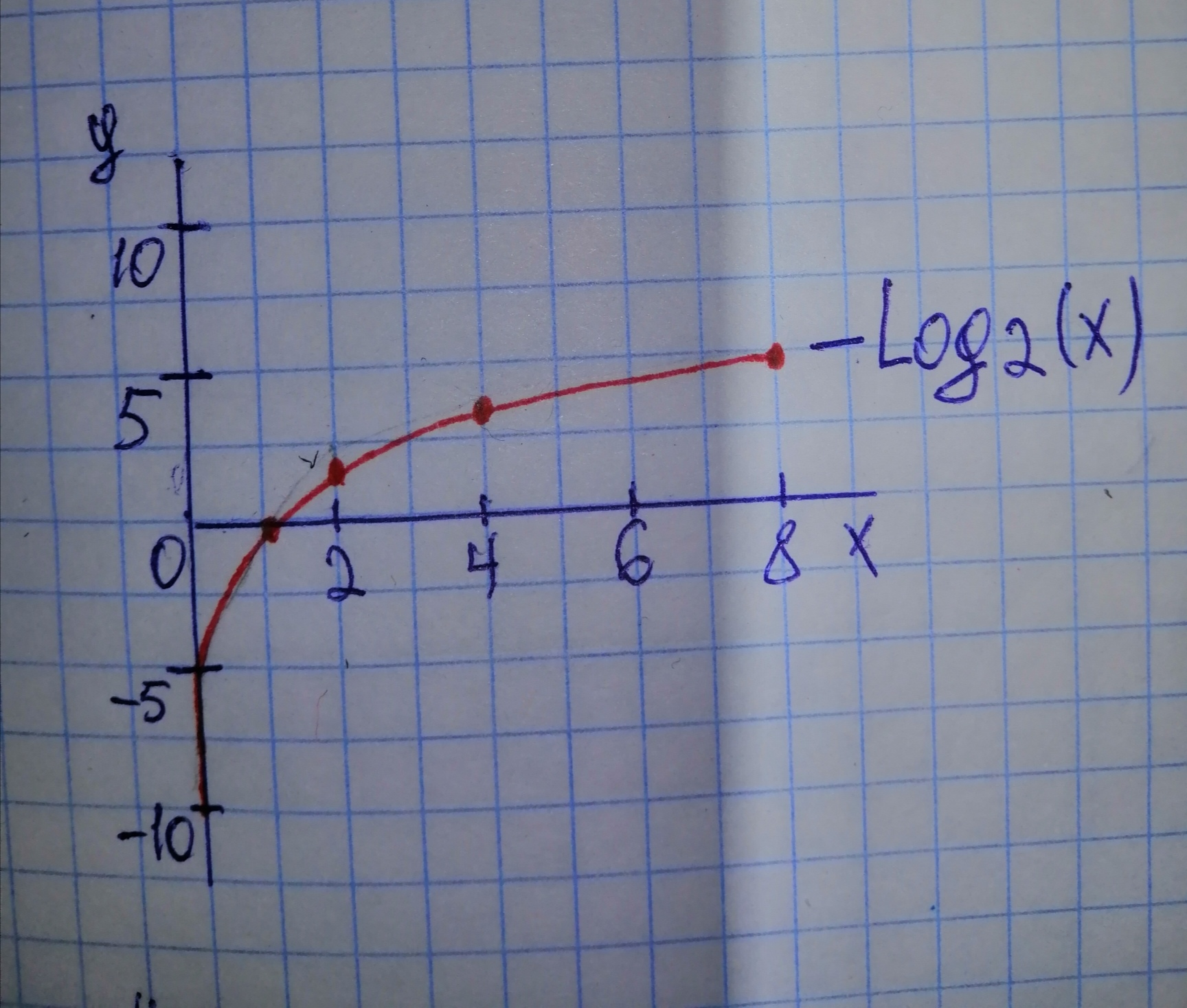# Begin by graphing f(x)=log_{2}x Then use transformations of this graph to graph the given function. What is the vertical asymptote? Use the graphs to determine each functions domain and range. g(x)= -2log_{2}xAlbarellak 2021-01-28 Answered
Begin by graphing $f\left(x\right)={\mathrm{log}}_{2}x$ Then use transformations of this graph to graph the given function. What is the vertical asymptote? Use the graphs to determine each functions domain and range.
You can still ask an expert for help

• Questions are typically answered in as fast as 30 minutes

Solve your problem for the price of one coffee

• Math expert for every subject
• Pay only if we can solve itbrawnyN

Step 1 For the following Logarithmic function defined by 1) Graphing Logarithmic function given in equation (1) requires setting up table of coordinates, so that

Step 2 We plot the following points between (x, y) determined from the table of coordinates and connect them with the continuous curve which represent the Logarithmic function $f\left(x\right)={\mathrm{log}}_{2}x$ as shown in Figure (1). Figure (1) represent the graph of Logarithmic functionStep 3 Note that: The y-axis which represented by the equation $x=0$ is the vertical asymptote, so that the curve approches, but never touches the positive portion of the y-axis as shown in figure (1). The domain of .

Step 4 To graph the Logarithmic function